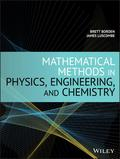John Wiley & Sons Mathematical Methods in Physics, Engineering, and ChemistryA concise and up-to-date introduction to mathematical methods for students in the physical sciences .. Product #: 978-1-119-57965-6 Regular price: \$122.86 \$122.86 Auf Lager

# Mathematical Methods in Physics, Engineering, and Chemistry1. Auflage Dezember 2019
448 Seiten, Hardcover
Wiley & Sons Ltd

ISBN: 978-1-119-57965-6
John Wiley & Sons

Jetzt kaufen

### Preis: 129,00 €

Preis inkl. MwSt, zzgl. Versand

A concise and up-to-date introduction to mathematical methods for students in the physical sciences

Mathematical Methods in Physics, Engineering and Chemistry offers an introduction to the most important methods of theoretical physics. Written by two physics professors with years of experience, the text puts the focus on the essential math topics that the majority of physical science students require in the course of their studies. This concise text also contains worked examples that clearly illustrate the mathematical concepts presented and shows how they apply to physical problems.

This targeted text covers a range of topics including linear algebra, partial differential equations, power series, Sturm-Liouville theory, Fourier series, special functions, complex analysis, the Green's function method, integral equations, and tensor analysis. This important text:
* Provides a streamlined approach to the subject by putting the focus on the mathematical topics that physical science students really need
* Offers a text that is different from the often-found definition-theorem-proof scheme
* Includes more than 150 worked examples that help with an understanding of the problems presented
* Presents a guide with more than 200 exercises with different degrees of difficulty

Written for advanced undergraduate and graduate students of physics, materials science, and engineering, Mathematical Methods in Physics, Engineering and Chemistry includes the essential methods of theoretical physics. The text is streamlined to provide only the most important mathematical concepts that apply to physical problems.

BRETT BORDEN, PHD, joined the Faculty of the Naval Postgraduate School in Monterey, CA in 2002, where he is Emeritus Professor of Physics. He served on the editorial board of the journal Inverse Problems from 2002 to 2013. Dr. Borden is a Fellow of The Institute of Physics.

JAMES LUSCOMBE, PHD, is a Professor at the Naval Postgraduate School in Monterey, California. He teaches a variety of topics, including general relativity, statistical mechanics, mathematical methods, and quantum computation.